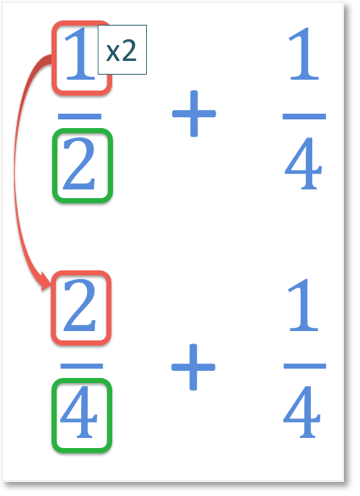# Adding Fractions with the Same Denominator

Adding Fractions with the Same Denominator• The denominator of a fraction is the number on the bottom of the fraction, below the dividing line.
• The denominator tells us how many equal parts that the item is divided into.
• Both fractions   2 / 4   and   1 / 4   have a denominator of four (These are quarters).
• The numerators are the numbers on top of each fraction, which represent how many parts there are.
• The fraction of   2 / 4   means that there are two out of four equal parts.
• The fraction of   1 / 4   means that there is one of these four equal parts.
• To add these fractions, the number of parts we are counting remains the same.
• We are counting the number of quarters there are and so the answer will also have a denominator of four.
• We can see that adding two quarters and one quarter is equal to 3 of these quarters.
• We add the numerators of the fractions to find the numerator in the answer.
To add fractions with the same denominators, the denominator remains the same and we add the numerators together.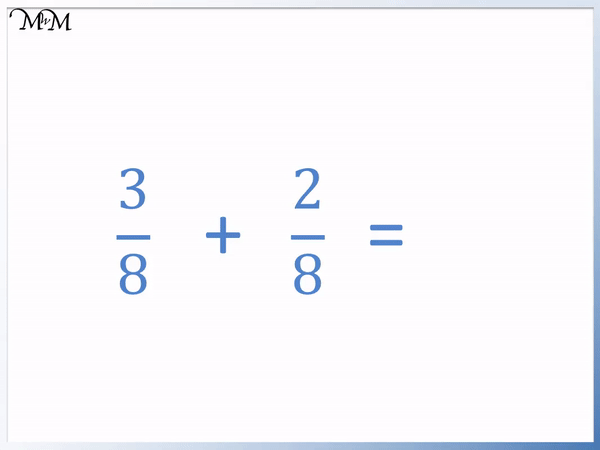• To add fractions with the same denominators, the denominator remains the same and we add the numerators together.
• The denominators are the numbers on the bottoms of the fractions and they are the same in both fractions that we are adding.
• The denominators of both fractions are ‘8’ and so the answer will also have a denominator of ‘8’.
• Now add the numerators, which are the numbers on the top of the fractions.
• 3 + 2 = 5.
• 3 / 8   +   2 / 8   =   5 / 8Supporting Lessons#### Adding Fractions with the Same Denominator: Interactive Questions

Adding Fractions with the Same Denominator: Interactive Questions

# How to Add Fractions with the Same Denominators

Steps for adding fractions with the same denominator:

1. The denominator of the answer is the same as the denominators in the fractions being added.
2. Add the numerators of the fractions to make the numerator of the answer.

These steps can be more easily interpreted to mean:

1. The number on the bottom of the fractions is kept the same.
2. Add the numbers on the tops of the fractions.

To understand how we add fractions with the same denominator and why this method works, we will begin by looking at an example.

Below, we have

12   +   12 .

The denominators are the numbers on the bottoms of the fractions. Both fractions have a denominator of ‘2’ and so, we are adding fractions with the same denominators.

When fractions have the same numbers on the bottom, they are said to have a common denominator. This is another way of saying that the fractions have the same denominators. They have a denominator in common.

The denominator tells us how many equal parts to divide a fraction into. A denominator of 2 means that the whole amount will be divided into 2 equal parts, or halves.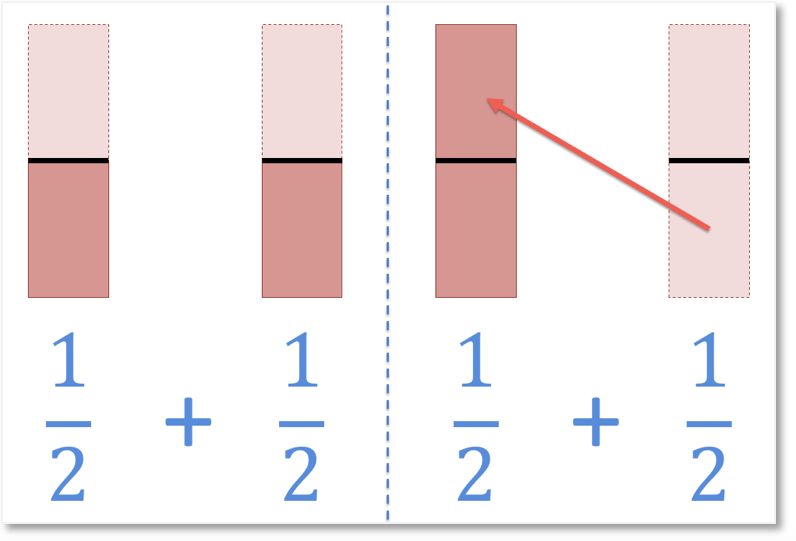If we take the half from the fraction on the right and combine it with the fraction on the left, we can see that the whole shape is now shaded in.

We have added the two fractions together to make a total.

We have    22    parts of the shape shaded in.

So, we can say that

12  +  12    =   22  .

To get this result, we kept the denominator, on the bottom of the fraction the same. The denominator was ‘2’.

We then added the numerators of the two fractions: ‘1’ + ‘1’ and made ‘2’.

Because the whole shape is shaded in, we can also say that

12   +  12    = 1.

22    is the same value as 1 whole and is just a simpler way to write it.

Another way to interpret this fraction is that we have two out of two equal parts. We have two halves.

Another way to interpret the fraction is to remember that the line between the numerator and denominator means to perform a division.

The fraction means 2 ÷ 2, which equals 1.

Below is another example of adding two fractions that have the same denominator.

The fractions of  24  and  14   both have the same digits on the bottom, below the dividing line. They have a common denominator of four.

Fractions with a denominator of 4 are describing a division into four equal parts. These are called quarters.

When adding these fractions, we can think of the process as counting up the total number of quarters that we have.

And since we are counting the number of quarters we have, the answer will be in quarters too.

The denominator in our answer will therefore be a ‘4’.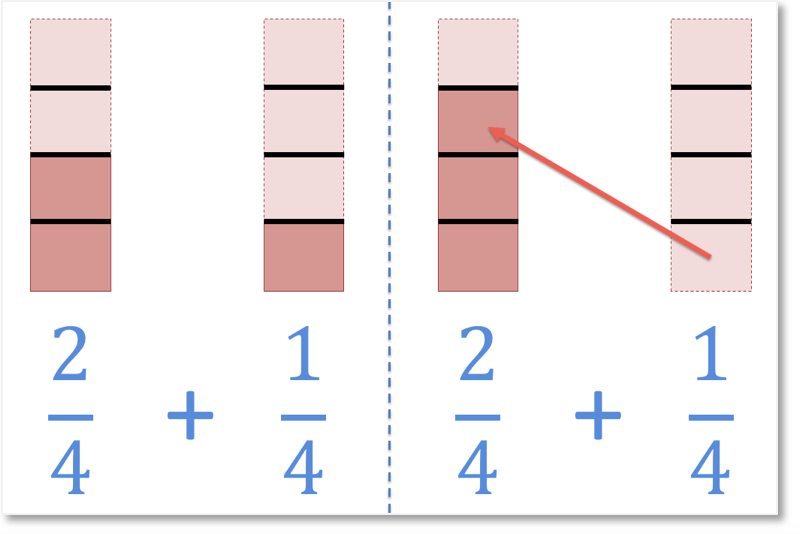In the example above we have:

24  +  14 .

Remember that the method for adding fractions with the same denominator is to keep the same denominator and add the numerators.

The denominator in the answer will be a ‘4’.

The numerators are ‘2’ and ‘1’. 2 + 1 = 3.

24  +  14  = 34  .

We have combined the quarters to make a total of three quarters.

The shapes were divided into four equal parts and we are counting how many of these four equal parts we have in total.

There are two parts shaded in on the left and there is one part shaded in on the right, we have a total of three parts shaded in. And so, 3 out of 4 parts are shaded in.

We will look at the following example without using any bar model diagrams.

We will follow the two steps in our method for adding fractions with the same denominator.

We keep the denominator in our answer the same as the denominators in the fractions that we are adding.The denominators of both   15   and   35   are both 5.

The denominators are the same.

This means that the denominator in our answer will also be 5.To add fractions with the same denominators, we simply add the numerators.

1 + 3 = 4.

Therefore,

15   +   35   =   45 .

Provided that the fractions that are being added have the same denominators, then this method can always be used to add the fractions.

Here is a final example, involving an answer that is an improper fraction.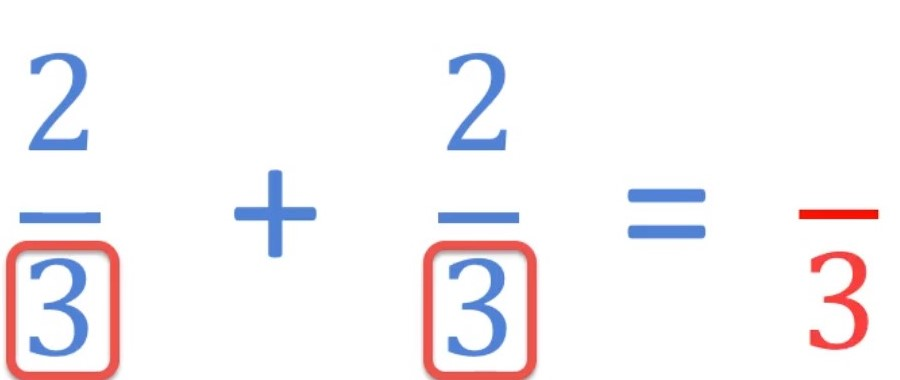The fractions being added are identical.

Both fractions are two-thirds.

23   and   23  both have a denominator of three.

Therefore the denominator in the answer will also be three.

To find the numerator of the fraction in the answer, add the numerators of the two fractions that you are adding.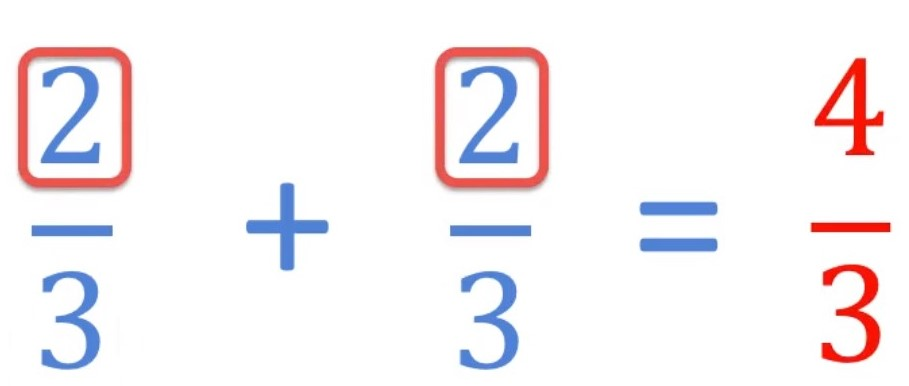2 + 2 = 4

Therefore:

23   +   23   =   43 .

When a fraction has a numerator larger than its denominator, it is known as an improper fraction.

An alternative name for an improper fraction is a top-heavy fraction.

It simply means that the number on the top of the fraction is larger than the number on the bottom of the fraction.

When adding fractions, you may obtain an answer that is an improper fraction.

The addition of the two fractions given in this example is really asking us ‘if we have two thirds and add two more thirds, how many thirds do we have in total’?The image above shows that we have four thirds shaded in. Each of the larger rectangles were divided equally into three parts and four of them are shaded in total.

One full rectangle is shaded, along with one extra third of a rectangle.

We have one whole and one third in total.

43 can be written as 1   13 .

When writing fractions it is often considered better to write the final answer as a whole number alongside a fraction, such as in this example.

A whole number alongside a fraction is called a mixed number.

When teaching adding fractions, it is best to start with this lesson on adding fractions that have a common denominator and mastering this skill before progressing onto adding fractions that have different denominators.

Only move on to adding or subtracting fractions with unlike denominators once this process is well-understood.

It is best to start by showing the process visually, using bar models such as in this lesson, or pizza slices. Eventually you want to be able to add two fractions with the same denominator quickly and mentally, without the aid of a diagram.

The most common mistake with this method is adding the denominators together along with the numerators.

It is worthwhile pointing this mistake out in advance and frequently reminding that the denominators remain the same and that it is just the numerators that we add.

It may help to return to the visual rectangular bar models to help remember this if this mistake occurs.Now try our lesson on Adding Fractions with Unlike Denominators where we learn the method for adding fractions that do not have the same denominators.